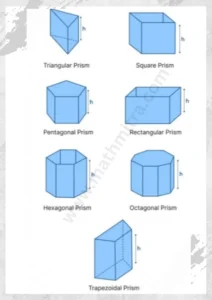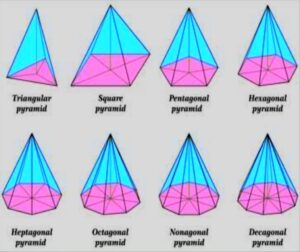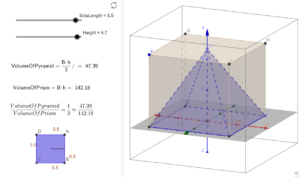December 10, 2023

# Prism and Pyramid

A prism is a three-dimensional geometric structure with two bases, which are often polygonal, and three or more lateral faces, which are rectangular in shape. A prism’s sides are all perpendicular to the bases, and its lateral faces are all parallelograms. The shape of a prism’s base determines its name. For instance, the base of a triangular prism is triangular, while the base of a rectangular prism is rectangular.

A pyramid is a three-dimensional geometric shape that has a base, which is usually a polygon, and triangular faces called lateral faces that meet at a single point called the apex. The sides of a pyramid are all triangles, and the lateral faces of a pyramid are all congruent isosceles triangles. Pyramids are also named based on the shape of their base. For example, a triangular pyramid has a triangular base, and a square pyramid has a square base.

## Difference between Prism and Pyramid

The main difference between a prism and a pyramid is the shape of their lateral faces. A prism has rectangular lateral faces, while a pyramid has triangular lateral faces. In addition, the lateral faces of a pyramid meet at a single point called the apex, while the lateral faces of a prism are not connected in this way.

Another difference between a prism and a pyramid is the way they are named. A prism is named based on the shape of its base, while a pyramid is named based on the shape of its base and the position of its apex. For example, a triangular pyramid has a triangular base and the apex is above the base, while a triangular prism has a triangular base but the lateral faces are rectangular.

Lastly, the volume of a prism can be calculated by multiplying the area of its base by its height, while the volume of a pyramid can be calculated by multiplying the area of its base by its height and dividing the result by 3.

### Different types of prism

There are several different types of prisms that are used in a variety of applications. Here are a few examples:

1. Triangular prism: This is a three-sided prism with two congruent triangular bases and three rectangular faces. It is used in a variety of optical applications, such as in binoculars or periscopes, to refract and reflect light.
2. Rectangular prism: This is a six-sided prism with two congruent rectangular bases and four rectangular faces. It is commonly used in geometry to study the properties of three-dimensional objects.
3. Pentagonal prism: This is a five-sided prism with two congruent pentagonal bases and five rectangular faces. It is used in some optical applications to refract and reflect light.
4. Hexagonal prism: This is a six-sided prism with two congruent hexagonal bases and six rectangular faces. It is used in a variety of applications, including in the construction of certain types of telescopes.
5. Rhombic prism: This is a six-sided prism with two congruent rhomboidal bases and four rectangular faces. It is used in some optical applications to refract and reflect light.
6. Dispersion prism: This is a triangular prism that is used to separate white light into its component colors. It is used in a variety of applications, including in spectroscopes and other scientific instruments.#### Different types of pyramid

There are several different types of pyramids that are distinguished based on the shape of their base and the number of faces they have. Here are a few examples:

1. Triangular pyramid: This is a pyramid with a triangular base and four triangular faces.
2. Square pyramid: This is a pyramid with a square base and four triangular faces.
3. Pentagonal pyramid: This is a pyramid with a pentagonal base and five triangular faces.
4. Hexagonal pyramid: This is a pyramid with a hexagonal base and six triangular faces.
5. Octagonal pyramid: This is a pyramid with an octagonal base and eight triangular faces.
6. Conical pyramid: This is a pyramid with a circular base and a pointed apex. It is also known as a cone pyramid.
7. Double pyramid: This is a pyramid with two bases, one on top of the other. It is also known as a bipyramid.
8. Triangular bipyramid: This is a double pyramid with two triangular bases.
9. Square bipyramid: This is a double pyramid with two square bases.
10. Pentagonal bipyramid: This is a double pyramid with two pentagonal bases.##### Volume of prism and pyramid :
• Volume of a prism =

We can fit the pyramid within the prism if we have a prism and a pyramid with equal bases and heights. In fact, when this is the case, the pyramid takes up exactly one-third of the space in the prism. This fact enables us to see a link between the volume of a prism and the volume of a pyramid when their bases and heights are equal. In other words, the volume of a pyramid is one-third that of a prism with identical height and base.

Let us now consider the volume of a pyramid. When their bases and heights are equal, a pyramid takes up 1/3 the volume of a prism. As a result, the volume of a pyramid is equal to 1/3 of the volume of a prism. So:

Comparing the Volume of Prisms and Pyramids:You might also be interested in:#### Bina singh

View all posts by Bina singh →
Last Minute Preparation Tips for SSC CGLTier II 2023 ssc chsl 2023 tier 1 cut off NIRF Rankings 2023 : Top 10 Engineering colleges in India CBSE Compartment Exam 2023 Application Form SSC CGL 2023 Notification: Important Dates